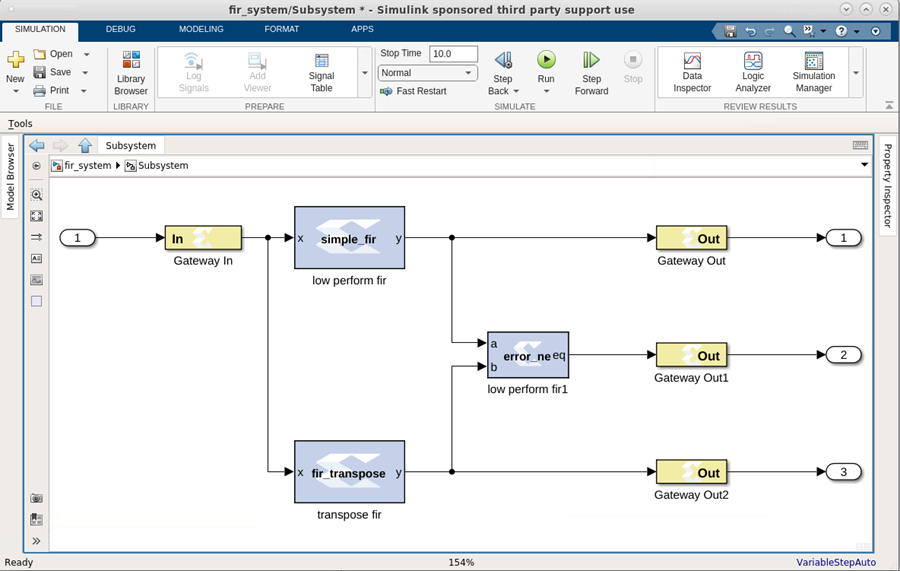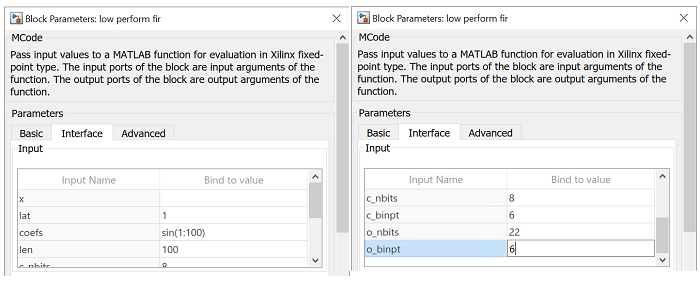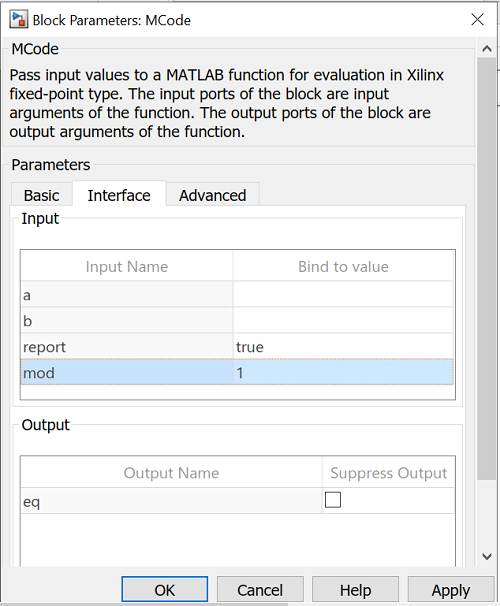# FIR Example and System Verification - 2022.1 English

## Vitis Model Composer User Guide (UG1483)

Document ID
UG1483
Release Date
2022-05-26
Version
2022.1 English

This example shows how to use the MCode block to model FIRs. It also shows how to do system verification with the MCode block.

Figure 1. FIR ExampleThe model contains two FIR blocks. Both are modeled with the MCode block and both are synthesizable. The following are the two functions that model those two blocks.

``````function y = simple_fir(x, lat, coefs, len, c_nbits, c_binpt, o_nbits, o_binpt)
coef_prec = {xlSigned, c_nbits, c_binpt, xlRound, xlWrap};
out_prec = {xlSigned, o_nbits, o_binpt};

coefs_xfix = xfix(coef_prec, coefs);
persistent coef_vec, coef_vec = xl_state(coefs_xfix, coef_prec);
persistent x_line, x_line = xl_state(zeros(1, len-1), x);
persistent p, p = xl_state(zeros(1, lat), out_prec, lat);

sum = x * coef_vec(0);
for idx = 1:len-1
sum = sum + x_line(idx-1) * coef_vec(idx);
sum = xfix(out_prec, sum);
end
y = p.back;
p.push_front_pop_back(sum);
x_line.push_front_pop_back(x);
function y = fir_transpose(x, lat, coefs, len, c_nbits, c_binpt, o_nbits, o_binpt)
coef_prec = {xlSigned, c_nbits, c_binpt, xlRound, xlWrap};
out_prec = {xlSigned, o_nbits, o_binpt};
coefs_xfix = xfix(coef_prec, coefs);
persistent coef_vec, coef_vec = xl_state(coefs_xfix, coef_prec);
persistent reg_line, reg_line = xl_state(zeros(1, len), out_prec);
if lat <= 0
error('latency must be at least 1');
end
lat = lat - 1;
persistent dly,
if lat <= 0
y = reg_line.back;
else
dly = xl_state(zeros(1, lat), out_prec, lat);
y = dly.back;
dly.push_front_pop_back(reg_line.back);
end
for idx = len-1:-1:1
reg_line(idx) = reg_line(idx - 1) + coef_vec(len - idx - 1) * x;
end
reg_line(0) = coef_vec(len - 1) * x;
``````

The parameters are configured as following:

Figure 2. ParametersIn order to verify that the functionality of the two blocks is equal, another MCode block is used to compare the outputs of the two blocks. If the two outputs are not equal at any given time, the error checking block will report the error. The following function performs the error checking:

``````function eq = error_ne(a, b, report, mod)
persistent cnt, cnt = xl_state(0, {xlUnsigned, 16, 0});
switch mod
case 1
eq = a==b;
case 2
eq = isnan(a) || isnan(b) || a == b;
case 3
eq = ~isnan(a) && ~isnan(b) && a == b;
otherwise
eq = false;
error(['wrong value of mode ', num2str(mod)]);
end
if report
if ~eq
error(['two inputs are not equal at time ', num2str(cnt)]);
end
end
cnt = cnt + 1;
``````

The block is configured as following:

Figure 3. Block Configuration